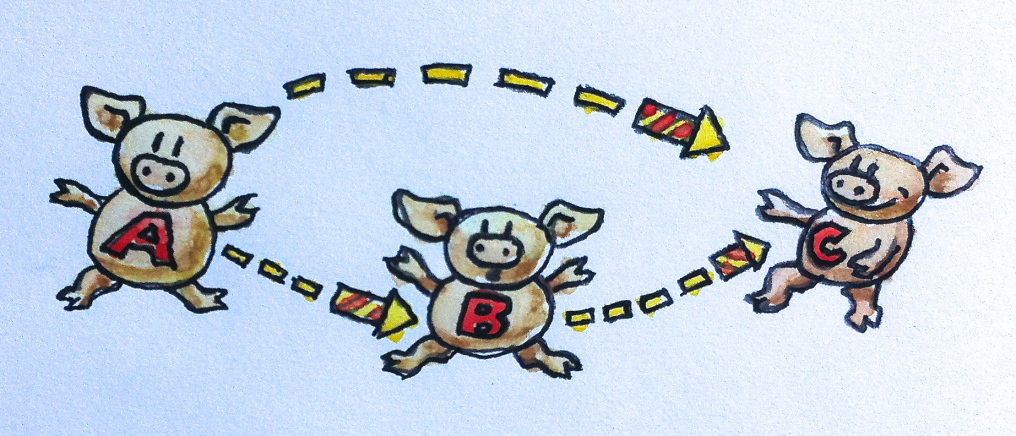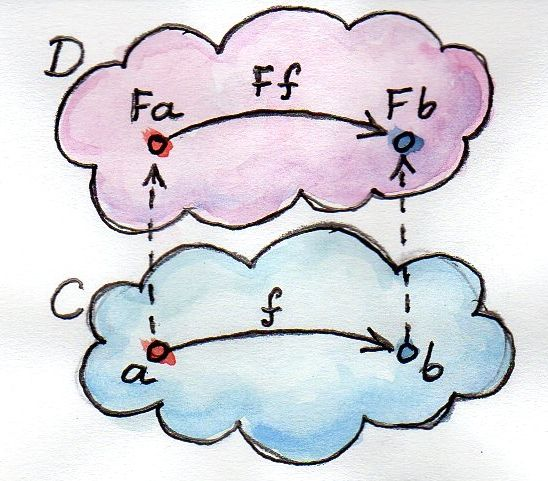#### High Order "Transformation" Function

##### ScalaIO 2018@dplaindoux

#### (→) : ∗ → (∗ → ∗)

Right Associativity

#### a → a

Identity Function aka id

id a ≝ a

id ≝ λ a . a abstraction

#### (a → b) → (a → b)

Application Function aka apply or \$

apply f a ≝ f a reification

apply f ≝ λ a . f a abstraction

apply f ≝ f η-reduction

apply ≝ λ f . f abstraction

apply id rewrite

#### (a → b) → (a → b)

High Order Function
"Transformation" Function

#### (b → c) → (a → b) → (a → c)

Composition Function aka

g f ≝ λ a . g (f a)
(f g) h ≝ λ a. f (g (h a))
f (g h) ≝ λ a. f (g (h x))
(f g) h ≡ f (g h)

#### (a → b) → (b → c) → (a → c)

Pipelining Function aka |>

f |> g ≝ g f
(f g) h ≡ f (g h)
(g |> f) h ≡ f (g h)
h |> (g |> f) ≡ f (g h)
h |> (g |> f) ≡ (g h) |> f
h |> (g |> f) ≡ (h |> g) |> f

#### ∀ m : ∗ → ∗

Abstraction over Type Constructors

#### (a → b) → (m a → m b)

Function Mapping aka fmap

#### functor ∀ m:∗ → ∗

• fmap :: (a → b) → (m a → m b)

#### a → m a

Lifting Function aka pure

#### m (a → b) → (m a → m b)

Applicative Functor aka <∗>

Given

• pure :: a → m a
• <∗> :: m (a → b) → (m a → m b)

fmap f ≝ ?

(a → b) → ?

fmap f ≝ f

(a → b) → (a → b)

fmap f ≝ pure f

(a → b) → m (a → b)

fmap f ≝ pure f <∗>

(a → b) → (m a → m b)

#### applicative ∀ m:∗ → ∗

• when functor m
• pure :: a → m a
• <∗> :: m (a → b) → (m a → m b)

#### m (m a) → m a

Flattening Function aka join

#### (a → m b) → (m a → m b)

Combining Computation Function aka bind

Given

• applicative m
• join :: m (m a) → m a

bind f ≝ ?

(a → m b) → ?

bind f ≝ f

(a → m b) → (a → m b)

bind f ≝ fmap f

(a → m b) → (m a → m (m b))

bind f ≝ fmap f |>

(a → m b) → (m (m b) → ?) → (m a → ?)

bind f ≝ fmap f |> join

(a → m b) → (m a → m b)

#### monad ∀ m:∗ → ∗

• when applicative m
• join :: m (m a) → m a
• bind :: (a → m b) → (m a → m b)

#### (a → m b) → (b → m c) → (a → m c)

Kleisli Monad Pipelining aka >=>

(f >=> g) >=> h ≡ f >=> (g >=> h)

Given

• monad m

f >=> g ≝ ?

(a → m b) → (b→ m c) → ?

f >=> g ≝ f

(a → m b) → (b→ m c) → (a → m b)

f >=> g ≝ f |>

(a → m b) → (b→ m c) → (m b → ?) → (a → ?)

f >=> g ≝ f |>
g :: b → m c
(a → m b) → (b→ m c) → (m b → ?) → (a → ?)

f >=> g ≝ f |>
bind g :: m b → m c
(a → m b) → (b→ m c) → (m b → ?) → (a → ?)

f >=> g ≝ f |> (bind g)

(a → m b) → (b→ m c) → (a → m c)

#### Master your types

 (a → m b) → (m a → m b) Function centric m a → (a → m b) → m b Data centric

Function centric approach promotes the composition

#### Draw your types#### Understand your types#### Going Further

• Pointfree i.e. Function as a Composition of Other Functions
• Category Theory and Declarative Programming by Bartosz Milewski
• Category: The Essence of Composition by Bartosz Milewski

#### Bind-Kleisli rewrite

```
(bind f) >=> g
≡ (bind f) |> (bind g)              -- rewrite
≡ (bind g) . (bind f)               -- rewrite
≡ a → (bind g) . (bind f) a         -- η-expansion
≡ a → bind g (bind f a)             -- rewrite
≡ a → bind (x → (bind g) (f x)) a   -- bind associativity
≡ a → bind (x → (bind g) . f x) a   -- rewrite
≡ a → bind ((bind g) . f) a         -- η-reduction
≡ a → bind (f |> (bind g)) a        -- rewrite
≡ bind (f |> (bind g))              -- η-reduction
≡ bind (f >=> g)                    -- rewrite
```

#### Kleisli associativity

```
(f >=> g) >=> r
≡ (f |> (bind g)) |> (bind r)       -- rewrite
≡ f |> ((bind g) |> (bind r))       -- Pipelining associativity
≡ f |> ((bind g) >=> r)             -- rewrite
≡ f |> (bind (g >=> r))             -- Bind-Kleisli rewrite
≡ f >=> (g >=> r)                   -- rewrite
```